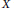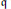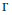Home

# Equivariant Map Queer Lie Superalgebras

## Abstract

An equivariant map queer Lie superalgebra is the Lie superalgebra of regular maps from an algebraic variety (or scheme)$X$ to a queer Lie superalgebra$\mathfrak{q}$ that are equivariant with respect to the action of a finite group$\Gamma$ acting on$X$ and$\mathfrak{q}$ . In this paper, we classify all irreducible finite-dimensional representations of the equivariant map queer Lie superalgebras under the assumption that$\Gamma$ is abelian and acts freely on$X$ . We show that such representations are parameterized by a certain set of$\Gamma$ -equivariant finitely supported maps from$X$ to the set of isomorphism classes of irreducible finite-dimensional representations of$\mathfrak{q}$ . In the special case where$X$ is the torus, we obtain a classification of the irreducible finite-dimensional representations of the twisted loop queer superalgebra.

## References

Hide All
MathJax
MathJax is a JavaScript display engine for mathematics. For more information see http://www.mathjax.org.

# Equivariant Map Queer Lie Superalgebras

## Metrics

### Full text viewsFull text views reflects the number of PDF downloads, PDFs sent to Google Drive, Dropbox and Kindle and HTML full text views.

Total number of HTML views: 0
Total number of PDF views: 0 *Loading metrics...

### Abstract viewsAbstract views reflect the number of visits to the article landing page.

Total abstract views: 0 *Loading metrics...

* Views captured on Cambridge Core between <date>. This data will be updated every 24 hours.

Usage data cannot currently be displayed.